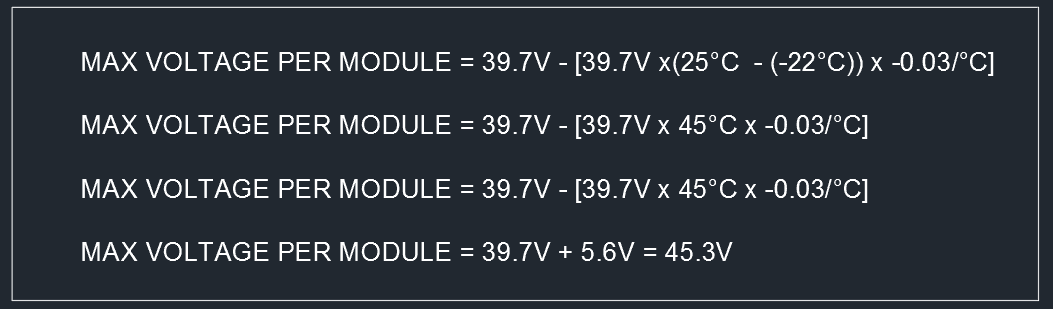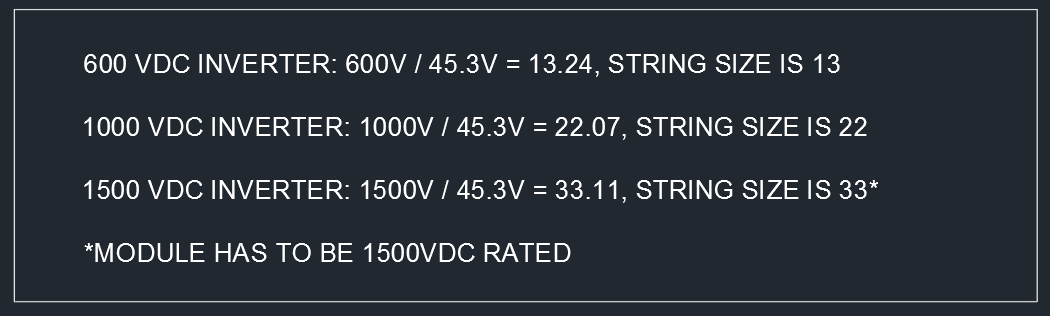# How to calculate the string size in a solar installation

I am going to explain how to calculate the string size of any PV system in any part of the world. All what you need is the data sheet of the solar module you are intending to use and the min temperature for the location of the installation.

In 2008, the National Electrical Code (NEC) added a second paragraph to 690.7(A) indicating, “When open-circuit voltage temperature coefficients are supplied in the instructions for listed PV modules, they shall be used to calculate the maximum PV system voltage as required by 110.3(B) instead of using Table 690.7.” I have tested 690.7 vs the method I am going to show, I got the same results down to -10ºC, but at temperatures lower than -10ºC,  the string size result is one module less than the method I am going to explain. One module can make a lot of difference when guarantying energy production.

First we calculate the Max voltage a single module will produce under extreme low temperature. The equation is shown in Fig.1Fig. 1 Max Voltage per module

Let’s assume we are using a 60 cel SolarWorld 285w mono module, which has a Voc = 39.7v, and a TC Voc = -0.30%/ºC, we can assume that we have a record low temperature of -22ºC. When using the equation in Fig.1 we get 45.3V as shown in Fig.2:Fig. 2 Max Voltage for SW 285W mono at -22C

The next step is to determine the string size. All we need to do is to divide the result in Fig.2 by the DC inverter voltage rating, I will show 3 scenarios in Fig. 3:Fig. 3 String Size

If you multiply the result calculated in Fig.2 (45.3V) by the string sizes shown on Fig.3 you can get the Maximum System Voltage on any of those scenarios.